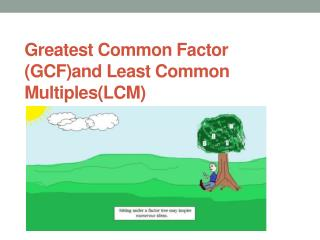DownloadDownload PresentationGreatest Common Factor (GCF)and Least Common Multiples(LCM)

# Greatest Common Factor (GCF)and Least Common Multiples(LCM)

Download Presentation## Greatest Common Factor (GCF)and Least Common Multiples(LCM)

- - - - - - - - - - - - - - - - - - - - - - - - - - - E N D - - - - - - - - - - - - - - - - - - - - - - - - - - -
##### Presentation Transcript

1. Greatest Common Factor (GCF)and Least Common Multiples(LCM)

2. What is the difference between a factor and a multiple?

3. Give me an example of a factor of 15

4. Give me an example of a multiple of 15

5. How would you find the GCF of 60 and 96?

6. There are actually 3 ways. You can use prime factorization, list all the prime factors for each number or extract common prime numbers.

7. List the factors of 60:1,2,3,4,5,6,10,12,15,20,30,60List the factors of 961,2,3,4,6,8,12,16,24,32,48,96find the largest factor - 12

8. …or do prime factorization. Circle all the primes the 2 numbers have in common and multiply one set of them to get your GCF. 96 60 48 2 2 30 24 2 15 2 12 2 3 5 6 2 2 x 2 x 3 = 12 2 3

9. …or you can extract the common prime factors of 60 and 96 and form a product to give the GCF. 2 60, 96 2 x 2 x 3 = 12 2 30, 48 3 15, 24 5, 8

10. Find the GCF (HCF) of 36, 24, 144 and 96

11. 96 24 36 48 2 2 12 18 2 24 2 6 9 2 2 12 2 3 2 3 3 6 2 144 2 3 12 12 3 4 2 x 2 x 3 = 12 3 4 2 2 2 2

12. 2 36, 24, 144, 96 2 x 2 x 3 = 12 2 18, 12, 72, 48 3 9, 6, 36, 24 3 2 12 8

13. There are 3 ways to find the LCM as well. You can list the multiples of the numbers, do prime factorization, or extract the prime factors from either one or both numbers.Find the LCM of 12 and 18

14. Multiples of 12 are…12,24,36,48,60,72,….Multiples of 18 are…18,36,54,72,90,108,…The smallest multiple the 2 numbers have in common is the least common multiple.

15. …or do prime factorization. Write down the number they have in common only once, then write down the leftover numbers. Multiply them all together. 12 18 9 4 2 3 3 3 2 2 Numbers in common are 2 and 3 Leftover numbers are 2 and 3 2 x 3 x 2 x 3 = 36

16. Remember…the numbers you pull out have to be prime numbers! 2 12, 18 2 6, 9 3 3, 9 3 1, 3 1 1 2 x 2 x 3 x 3 = 36

17. Find the LCM of 35, 420 and 245

18. 245 35 420 42 5 49 10 5 7 5 7 7 2 6 7 2 3 Numbers they have in common: 5 and 7 Leftover numbers: 2, 3, 2, 7 Multiply them all together: 5 x 7 x 2 x 3 x 2 x 7 = 2940

19. primes 5 35, 420, 245 7 7, 84, 49 7 1, 12, 7 3 1, 12, 1 2 1, 4, 1 2 1, 2, 1 1, 1, 1 5 x 7 x 7 x 3 x 2 x 2 = 2940

20. Find the GCF and LCM of 28 and 63

21. Find the GCF and LCM of12 and 13

22. Find the GCF and LCM of 27, 21, 33 and 15

23. GCF and LCM Problem Solving How can you tell if a word problem requires you to use Greatest Common Factor or Least Common Multiple to solve?

24. GCF Example: Applying what we have learned… • Samantha has two pieces of cloth. One piece is 72 inches wide and the other piece is 90 inches wide. She wants to cut both pieces into strips of equal width that are as wide as possible. How wide should she cut the strips?

25. LCM Example: Applying what we have learned… • Ben exercises every 12 days and Isabel every 8 days. Ben and Isabel both exercised today. How many days will it be until they exercise together again?

26. Question #1 • Mrs. Evans has 120 crayons and 30 pieces of paper to give to her students. What is the largest # of students she can have in her class so that each student gets equal # of crayons and equal # of paper.

27. Question #2 • Rosa is making a game board that is 16 inches by 24 inches. She wants to use square tiles. What is the larges tile she can use?

28. Question #3 • Z100 gave away a Z \$100 bill for every 100th caller. Every 30th caller received free concert tickets. How many callers must get through before one of them receives both a coupon and a concert ticket?

29. Question #4 • Two bikers are riding a circular path. The first rider completes a round in 12 minutes. The second rider completes a round in 18 minutes. If they both started at the same place and time and go in the same direction, after how many minutes will they meet again at the starting point?

30. Question #5 • Sean has 8-inch pieces of toy train track and Ruth has 18-inch pieces of train track. How many of each piece would each child need to build tracks that are equal in length?

31. Question #6 • I am planting 50 apple trees and 30 peach trees. I want the same number and type of trees per row. What is the maximum number of trees I can plant per row?